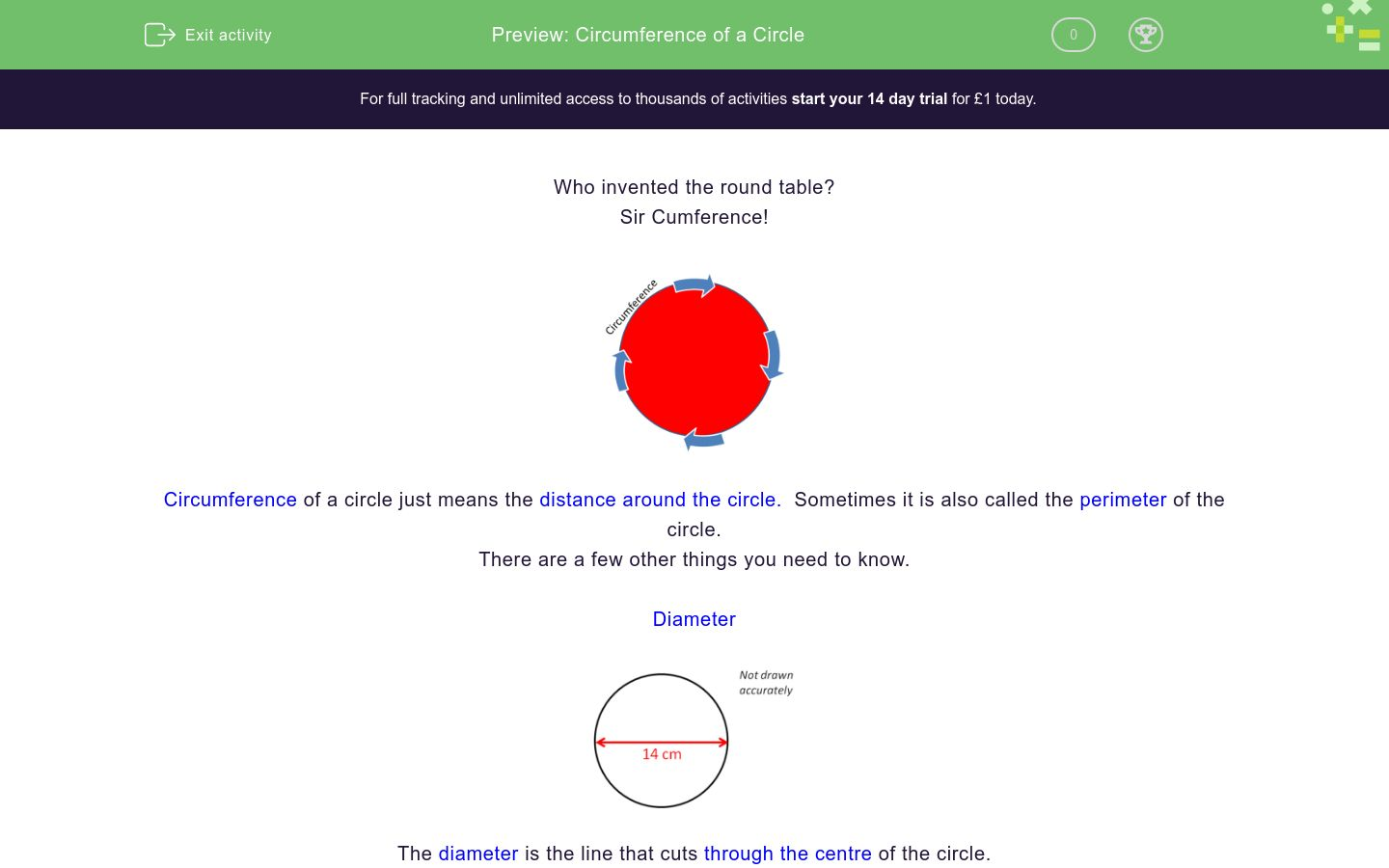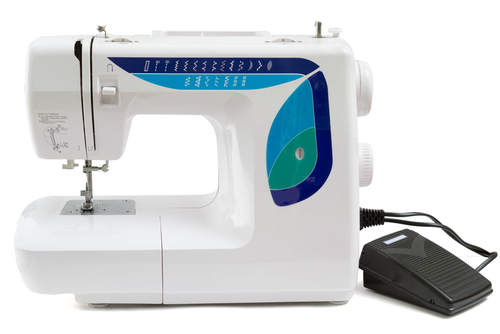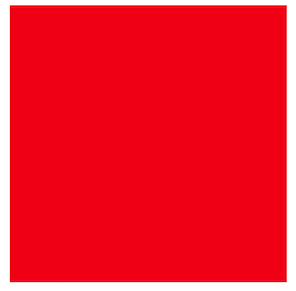# Circumference of a Circle

In this worksheet students will be able to calculate the circumference of a circle using pi and use their skill to solve simple problems.Key stage:  KS 4

GCSE Subjects:   Maths

GCSE Boards:   AQA, Eduqas, Pearson Edexcel, OCR

Curriculum topic:   Geometry and Measures, Mensuration

Curriculum subtopic:   Mensuration and Calculation, Perimeter Calculations

Difficulty level:### QUESTION 1 of 10

Who invented the round table?

Sir CumferenceCircumference of a circle just means the distance around the circle.  Sometimes it is also called the perimeter of the circle.

There are  a few other things you need to know.

DiameterThe diameter is the line that cuts through the centre of the circle.The radius comes from the centre of the circle to the edge and is half the diameter.

PiNo not that pie this one.You will need to learn the value for this..sorry. Did you know pi is infinite, some people can quote it to hundreds of decimal places.

I don't suppose they have Netflix.  Lucky for you that you only need to use it to 3 decimal places which is 3.142

Finally you need the formula for finding the circumference of a circle

circumference of a circle  = Pi x diameter  or C = pi x d

A great way to remember it is Cherry Pies are deliciousBuilding a tool box is useful.  Builders have them so do mathematicians as we are building our knowledge.

Our tool box contains definitions for Circumference, Perimeter, Diameter, Radius, Pi and the Formula. Looking good.Lets get startedCalculate the circumference of this circle.

Pi x diameter

3.142 x 14 = 43.988 cm  (42.99cm to 2 decimal places)

You will be asked to round to decimal places or significant figures.

WarningYou may get a question that gives you the radius.

Remember  the radius is only half of the diameter so double it first.

Calculate the circumference of a circle with a radius of 3 cm.

3 + 3 = 6 cm diameter

Now apply the formula Pi x diameter. 3.142 x 6 = 18.852  (18.9 to 3 significant figures)

Before you start the activities it is useful to know something.The diameter of this circle is 7 cm.  What is is circumference?

21.96 cm

21.98 cm

21.99 cm

22.00 cmThis circular pond has a  diameter of 6m.  What is its circumference?

Match up.  All answers have been rounded to 2 decimal places (2 d.p)

## Column B

Circumference with a diameter of 9 cm
34.56 cm
Circumference with a diameter of 2.5cm
16.34cm
Circumference with a radius of 2cm
28.28 cm
Circumference with a diameter of 11 cm
7.86 cm
Circumference with a radius of 1.7 cm
12.57 cm
Circumference with a radius of 2.6 cm
10.68 cmWhat are the  correct and incorrect calculations required to find the circumference of both circles?

 correct incorrect 3.142 x 18 3.142 x 6 3.142 x 15 3.142 x 12 3.142 x 6What is the perimeter of this semi circle with a diameter of 4 cm?

3.28 cm

6.28 cm

8.28 cm

10.28 cmA seamstress makes a circular table cloth with a diameter of 56 cm.

3.28 cm

6.28 cm

8.28 cm

10.28 cmFarmer Hay decided to build a semi circular cattle pen out of fencing with a brick wall forming the straight side.

If the radius of the semi circle is 10 m, how much fencing does Farmer Hay require?This semi circle and square two are going to be sewn together to make the shape of an arch.

An edging will then be sewn to the arch.

The square has side of 6.5 cm.

How much edging will be used?

24.71 cm

20.425 cm

24.92 cm

29.71 cmA boy runs round an athletics track consists of two semi-circles with a diameter of 60 metres, joined together by two straight sections, each with length 120 m.

Calculate the length of the one complete run around the athletics track.A sailing lake is shaped like a quarter circle.  The diameter is 800 m.  How long would a footpath be around the lake?

1256.40 metres

628.40 metres

2228.40 metres

1428.40 metres

• Question 1The diameter of this circle is 7 cm.  What is is circumference?

21.99 cm
EDDIE SAYS
Did your remember the formula, or cherry pies are delicious? This is quite straight forward really. 3.142 x 7 cm = 21.99 cm The care here is rounding it to the unit of measurement specified.
• Question 2This circular pond has a  diameter of 6m.  What is its circumference?

18.85 cm
EDDIE SAYS
3.142 x 6 = 18.852 (18.85 to 2 d.p) This calculation is useful to know to buy fencing for the pond to stop your little brother falling in.
• Question 3

Match up.  All answers have been rounded to 2 decimal places (2 d.p)

## Column B

Circumference with a diameter of ...
28.28 cm
Circumference with a diameter of ...
7.86 cm
Circumference with a radius of 2...
12.57 cm
Circumference with a diameter of ...
34.56 cm
Circumference with a radius of 1...
10.68 cm
Circumference with a radius of 2....
16.34cm
EDDIE SAYS
How did you go? Did you remember to double the radius to get the diameter on some questions. The answers were either 3.142 x diameter or double the radius first and then multiply by 3.142
• Question 4What are the  correct and incorrect calculations required to find the circumference of both circles?

 correct incorrect 3.142 x 18 3.142 x 6 3.142 x 15 3.142 x 12 3.142 x 6
EDDIE SAYS
Sometimes a little trickery goes on. Just testing you are not asleep. To find the circumference of the green circle you have only got the radius so double it first to get 18. 3.142 x 18 The white circle has give a radius of 6. 2 x 6 = 12 3.142 x 12
• Question 5What is the perimeter of this semi circle with a diameter of 4 cm?

10.28 cm
EDDIE SAYS
This is the type of question that can catch us out. Note the wording asked for perimeter. 3.142 x 4 = 12.568 As we want a semi circle we need to cut the answer in half 12.568 ÷2; = 6.284 (6.28) to 2 d.p This is where you need to visit that tool box and know that perimeter means around the whole of the shape. Half the circumference only gives the outer part of the shape You need to add the perimeter back in. 6.28 + 4 = 10.28 cm And no, it is not a trick question, there is no such thing.
• Question 6A seamstress makes a circular table cloth with a diameter of 56 cm.

EDDIE SAYS
3.142 x 56cm = 175.95 cm 56 ÷ 2 = 28 3.142 x 28 =87.98 cm You you see where this calculation can be required for may things?
• Question 7Farmer Hay decided to build a semi circular cattle pen out of fencing with a brick wall forming the straight side.

If the radius of the semi circle is 10 m, how much fencing does Farmer Hay require?

31.42 metres
EDDIE SAYS
3.142 x 20 = 62.84 62.84 ÷ 2 = 31.42 This is the type of question you can often skim over and come unstuck. By now you will have spotted that you need to double the radius to get the diameter before multiplying up and dividing by 2. However as the cattle pen has a brick wall as the straight side no fencing is required here. As in the previous semi circle question there is no need to add the straight side back in.
• Question 8This semi circle and square two are going to be sewn together to make the shape of an arch.

An edging will then be sewn to the arch.

The square has side of 6.5 cm.

How much edging will be used?

29.71 cm
EDDIE SAYS
This may look trickier than it actually is. It is just to get you thinking to solve problems. You can do it so just apply what you know. If the side of the square is 6.5cm, then this has to be the diameter of the circle too. To find the edging of the semi circle 3.142 x 6.5 = 20.42 20.42 ÷ 2 = 10.21 Now add three lots of 6 .5 for the remaining sides of the square. 10.21 + 19.5 = 29.71 You only need three sides of the square because one is sewn to the bottom of the semi circle.
• Question 9A boy runs round an athletics track consists of two semi-circles with a diameter of 60 metres, joined together by two straight sections, each with length 120 m.

Calculate the length of the one complete run around the athletics track.

308.52 metres
EDDIE SAYS
I always draw things out from a pure description as it helps to visualise what is asked for. 3.142 x 60 = 188.52. You don't have to halve this as their are two semi circles, which if you pushed together would make a whole circle. Now add on the two sides of 120m. 188.52m + 120m + 120 m =308.52 m
• Question 10A sailing lake is shaped like a quarter circle.  The diameter is 800 m.  How long would a footpath be around the lake?

1428.40 metres
EDDIE SAYS
Nothing to worry about here. You have been using all the skills in your tool kit up until now. 3.142 x 800m = 2513.60 metres 2513.60 ÷ 4 = 628.40 metres This gives us the outside part of the lake. We need to add in the straight sides. Be careful, we were given the diameter in the question but the sides are the radius of the circle. 628.40 metres + 400 + 400 = 1428.40 metres Did you remember to add on two lots of 400 metres?
---- OR ----

Sign up for a £1 trial so you can track and measure your child's progress on this activity.

### What is EdPlace?

We're your National Curriculum aligned online education content provider helping each child succeed in English, maths and science from year 1 to GCSE. With an EdPlace account you’ll be able to track and measure progress, helping each child achieve their best. We build confidence and attainment by personalising each child’s learning at a level that suits them.

Get started